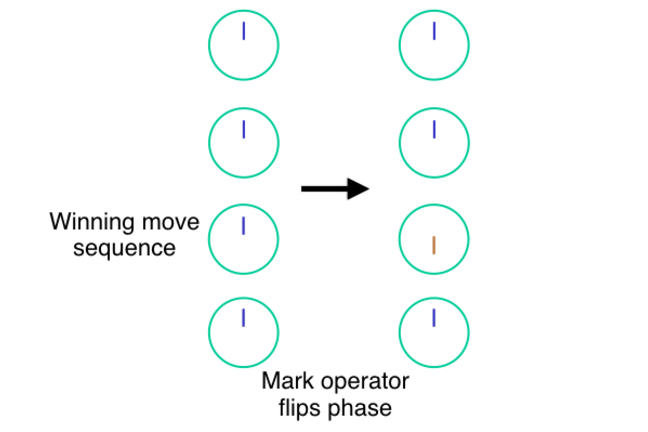3.3

## Keio University# Marking Desired States

Grover’s algorithm consists of two parts: marking and diffusion. First, we will discuss a little more formally the structure introduced in the previous Step, then we will see some examples of mark routines in this Step, and discuss diffusion and performance in the next Step.

## Overview of the Algorithm

Start with a function $f(x)$ that takes some input $x$ and returns TRUE or FALSE. Your goal is to find a value of $x$ for which it returns true. (For the moment, assume there is only one value for $x$ that returns true, although that doesn’t necessarily have to be so.) For our purposes here, assume that the characteristics of the problem are such that the number of possible values for $x$ is large, and you have no way of making an informed guess what might be a good value for $x$. Even if you calculate $f(x)$, it only gives you true/false, no “that was close” or anything that guides you toward a better guess for $x$ next time. All you can do is keep trying different values for $x$.

These kinds of problems are often called randomized database search problems, though I (Van Meter) am not wild about this particular term. We can rephrase them to the question, “For what value of $x$ does $f(x) = k$?” Unstructured problems like this are difficult to solve efficiently. With no ability to direct your search, on average, you would expect try half of the possibilities for $x$ until one returns true.

Grover’s algorithm allows you to perform the calculation of that function fewer times. If $N$ is the number of possible values for $x$, you must calculate $f(x)$ only $O(\sqrt{N})$ times, and it will give you your answer with >50% probability (usually close to 100%).

Of course, in accordance with our basic approach to quantum algorithms, this effect is achieved using interference, which depends on the phase of the states. Lov Grover’s fundamental insight is that it’s possible to implement an “inversion about the mean” operation that creates this interference, which we will see in the next Step.

To achieve this, we need two routines: a mark routine that labels our answers as good or bad, and a diffusion operator that “smears out” our probability amplitude over the other dials. The exact effect of that diffusion depends on whether or not that state was marked.

## The Mark Routine

The mark routine tests whether or not we have found the answer to our question. Of course it is implemented using the unitary gates we have already described, but this is largely a task with a simple classical analog, calculating $f(x)$ and returning true or false.

In our chess example, $x$ might be a sequence of moves, and $f(x)$ a function that returns true if the sequence results in a win and false if it results in a loss. For argument’s sake, let’s assume there are four possible moves, and exactly one of them results in checkmate (a win for us).

To find the best move classically, in the worst case we might have to try four times before hitting the win, and the average number is 2.5 times. If we use a quantum computer with two qubits and Grover’s search algorithm, we can find the answer using our check operation only one time.

To start the algorithm, we set up a superposition of all four states. (To encode four states using qubits, of course we use two qubits, so that the numbers $00$, $01$, $10$, and $11$ each represent one of our move sequences.) In our dial notation, that means our register $|x\rangle$ has four dials, each with the same amplitude and phase. This initialization gives us the set of four dials on the left in the diagram at the top of this article. (Again here, we are not using exactly correct vector lengths in the dials, in order to keep them visually easy to understand.)

Second, we execute our mark routine, which flips the phase (changes the phase by $\pi$) of only states where $f(x)$ is true. This is the transition corresponding to the arrow in the diagram. The state corresponding to the winning move sequence, shown in orange, now points down, opposite the phase of the three losing moves.

It’s important to note here that we can construct a circuit using the gates we have already described to make our function $f$ even if we do not know the answer; the function must know how to recognize an answer, but does not include that information directly. Otherwise the algorithm would just be confirming what we already know!